Next: A list of NPO Up: Introduction Previous: Approximate algorithms and approximation   Index

### Completeness in approximation classes

In this section we define a natural approximation preserving reducibility and introduce the notion of completeness both in NPO and in APX.

Definition 8

Let A and B be two NPO problems. A is said to be PTAS-reducible to B, in symbols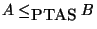, if three functions f, g, and c exist such that:

1.
For any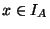and for any rational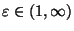,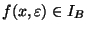is computable in time polynomial with respect to |x|.

2.
For any, for any, and for any rational,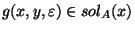is computable in time polynomial with respect to both |x| and |y|.

3.is computable and invertible.

4.
For any, for any, and for any rational,Remark 1

In  a different kind of reducibility between optimization problems is defined which is called L-reducibility. It is possible to prove that, within APX, the L-reducibility is a restriction of the PTAS-reducibility . More recently, another restriction of the PTAS-reducibility, called E-reducibility, has been introduced in .

Proposition 1   Ifand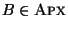(respectively,), then(respectively,).

Definition 9

A problem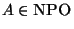is NPO-complete if, for any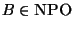,.

Definition 10

A problemis APX-hard if, for any,. An APX-hard problem is APX-complete if it belongs to APX.

From Proposition 1 it immediately follows that if an NPO problem A is NPO-complete (respectively, APX-hard) then it does not belong to APX (respectively, PTAS). It is also possible to prove that if A is NPO-complete (respectively, NPO PB-complete) then it cannot be approximated within(respectively,) for some.

The syntactically defined classes MAX SNP (containing e.g. MAXIMUM 3-SATISFIABILITY and MAXIMUM CUT) and MAX NP (containing e.g. MAXIMUM SATISFIABILITY) were defined in . Recently was shown that the closures of these classes under PTAS-reduction were identical to APX  and . In the compendium we therefore always use the term APX-complete instead of MAX SNP-complete and APX-hard instead of MAX SNP-hard.

The classes MAX PB and MIN PB consisting of the polynomially bounded maximization and minimization problems, respectively, were defined in . The closures of these classes under PTAS-reduction have recently been shown to be identical to NPO PB . In the compendium we therefore always use NPO PB-complete instead of MAX PB-complete and MIN PB-complete.Next: A list of NPO Up: Introduction Previous: Approximate algorithms and approximation   Index
Viggo Kann
2000-03-20